Related Articles
Access the elements of a Series in Pandas
• Last Updated : 06 Dec, 2018

Pandas Series is a one-dimensional labeled array capable of holding data of any type (integer, string, float, python objects, etc.). Labels need not be unique but must be a hashable type.

Let’s discuss different ways to access the elements of given Pandas Series.

First create a Pandas Series.

 `# importing pandas module ``import` `pandas as pd ``   ` `# making data frame ``df ``=` `pd.read_csv(``"https://media.geeksforgeeks.org/wp-content/uploads/nba.csv"``) `` ` `ser ``=` `pd.Series(df[``'Name'``])``ser.head(``10``)``# or simply df['Name'].head(10)`

Output: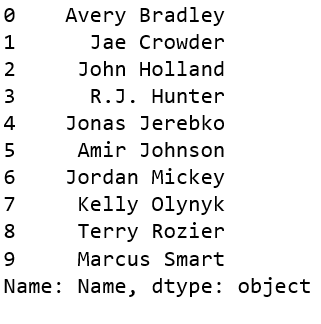Example #1: Get the first element of series

 `# importing pandas module ``import` `pandas as pd ``   ` `# making data frame ``df ``=` `pd.read_csv(``"https://media.geeksforgeeks.org/wp-content/uploads/nba.csv"``) `` ` `df[``'Name'``].head(``10``)`` ` `# get the first element``ser[``0``]`

Output: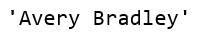Example #2: Access multiple elements by providing position of item

 `# importing pandas module ``import` `pandas as pd ``   ` `# making data frame ``df ``=` `pd.read_csv(``"https://media.geeksforgeeks.org/wp-content/uploads/nba.csv"``) `` ` `df[``'Name'``].head(``10``)`` ` `# get multiple elements at given index``ser[[``0``, ``3``, ``6``, ``9``]]`

Output: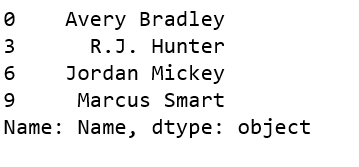Example #3: Access first 5 elements in Series

 `# importing pandas module ``import` `pandas as pd ``   ` `# making data frame ``df ``=` `pd.read_csv(``"https://media.geeksforgeeks.org/wp-content/uploads/nba.csv"``) `` ` `df[``'Name'``].head(``10``)`` ` `# get first five names``ser[:``5``]`

Output: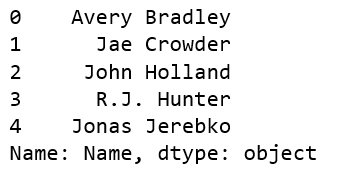Example #4: Get last 10 elements in Series

 `# importing pandas module ``import` `pandas as pd ``   ` `# making data frame ``df ``=` `pd.read_csv(``"https://media.geeksforgeeks.org/wp-content/uploads/nba.csv"``) `` ` `df[``'Name'``].head(``10``)`` ` `# get last 10 names``ser[``-``10``:]`

Output: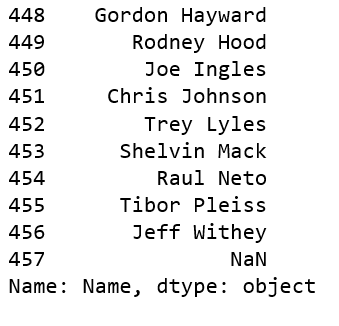Example #5: Access multiple elements by providing label of index

 `# importing pandas module ``import` `pandas as pd ``import` `numpy as np`` ` `ser ``=` `pd.Series(np.arange(``3``, ``15``), index ``=` `list``(``"abcdefghijkl"``))`` ` `ser[[``'a'``, ``'d'``, ``'g'``, ``'l'``]]`

Output: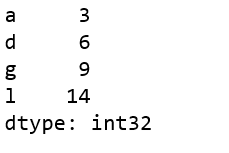Attention geek! Strengthen your foundations with the Python Programming Foundation Course and learn the basics.

To begin with, your interview preparations Enhance your Data Structures concepts with the Python DS Course.

My Personal Notes arrow_drop_up